The Federation is the oldest and only Jewish Women's
Federation in the Country - Since 1916

*ALL MEMBERS OF OUR CONSTITUENT ORGANIZATIONS
ARE MEMBERS OF FEDERATION

<<<<<<<<<<<<<<<<<<<<<<<<<<<<<<<<<<<<<<<<<<<<<<<<<<<<<<<<<<<Visit and Like us on Facebook to Stay Informed!

<<<<<<<<<<<<<<<<<<<<<<<<<<<<<<<<<<<<<<<<<<<<<<<<<<<<<<<<<<<

107th Annual Convention
Thursday May 18, 2023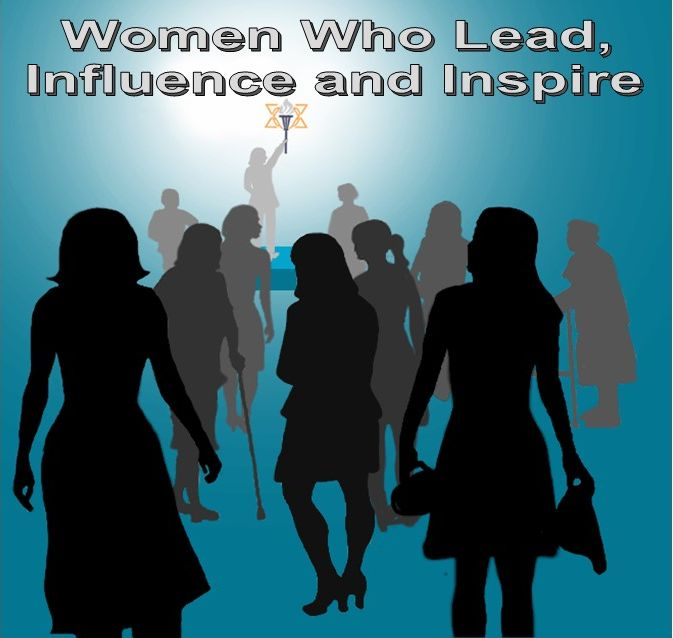Inspire and Influence

Keynote speaker: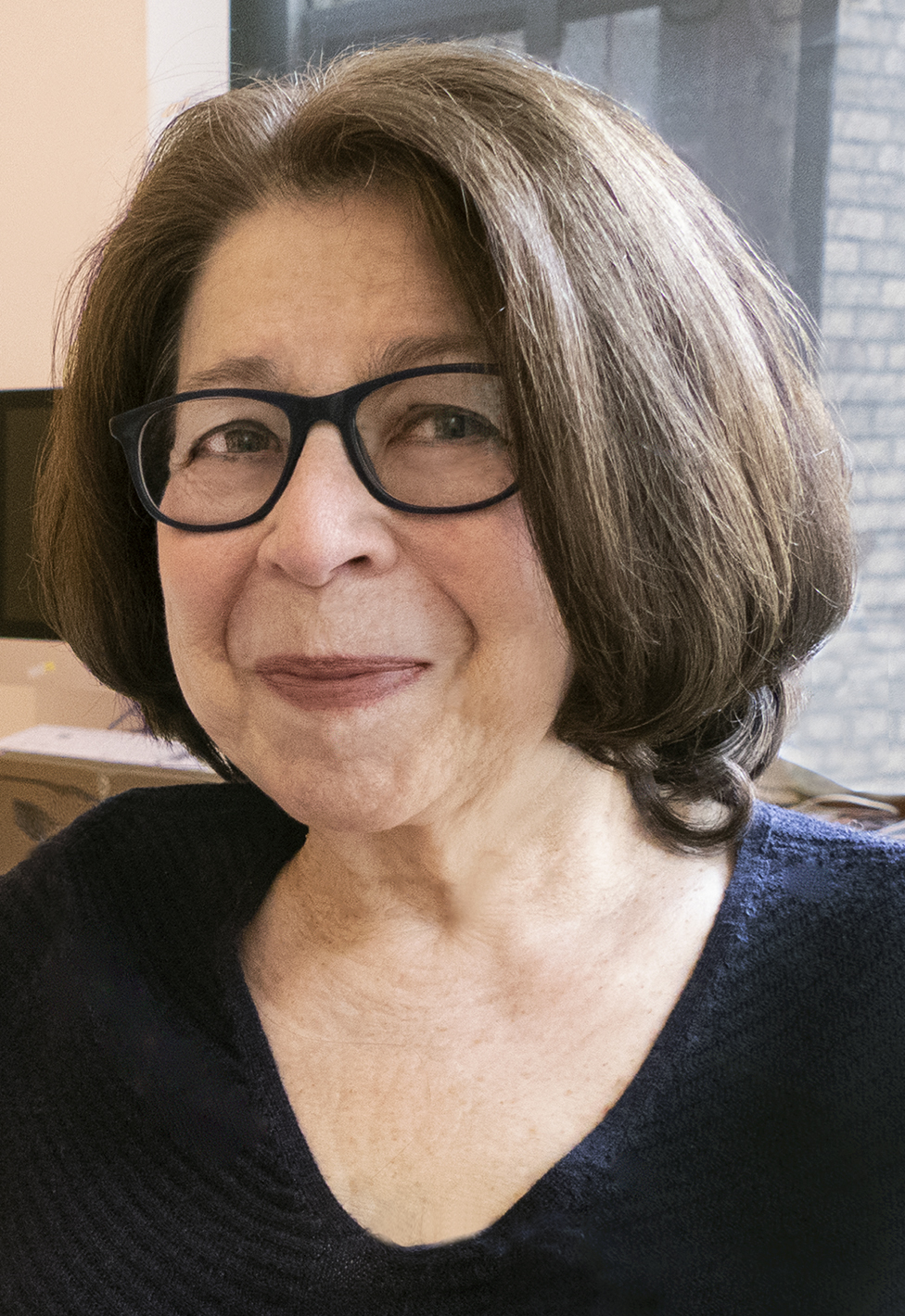Susan Weidman Schneider, co-founder and editor-in-chief of
Lilith Magazine

E.B Hirsh Achievement Award Recipient: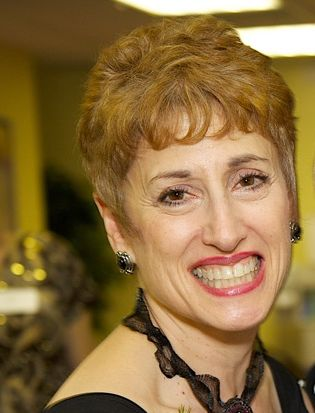Betsy Narrow,

"A Woman Who Leads the Way"
Award Honorees
Visit Award page to see our
2023 Award Honorees

Mazel Tov Honoree Form

<<<<<<<<<<<<<<<<<<<<<<<<<<<<<<<<<<<<<<<<<<<<<<<<<<<<<<<<<<<

What is Serv-A?

<<<<<<<<<<<<<<<<<<<<<<<<<<<<<<<<<<<<<<<<<<<<<<<<<<<<<<<<<<<

President, CeCe Rund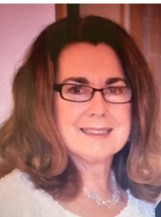<<<<<<<<<<<<<<<<<<<<<<<<<<<<<<<<<<<<<<<<<<<<<<<<<<<<<<<<<<<

Officers for 2023
President - CeCe Rund
Vice President - Julie Bernstein
Vice President - Shulamit Finkelstein
Vice President - Edie Meyers
Corresponding Secretaries - Rochelle Bohrer, Ferne Rogow
Recording Secretary - Eve Kresin Steinberg
Treasurer - Ann Fishkin
Auditor - Rosalind Asch
Community Representative - Marcia Bornfirend, Past President

<<<<<<<<<<<<<<<<<<<<<<<<<<<<<<<<<<<<<<<<<<<<<<<<<<<<<<<<<<<

 The Federation of Jewish Women's Organizations of Maryland is the Oldest Jewish Women's Federation in the Country Founded 1916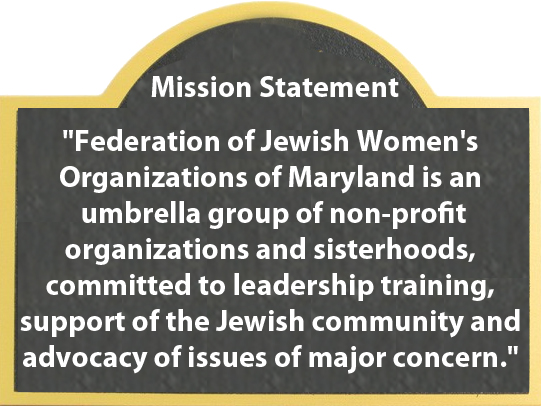Calendar To see the full calendar and Jewish holidays, CLICK HERE.